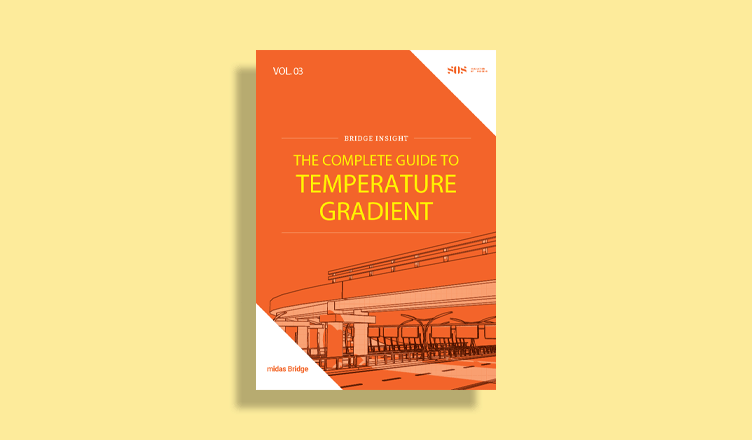BLOG BRIDGE INSIGHT

Steel Composite Girder Flexural Capacity: AASHTO vs Eurocode

## 1. Introduction

Steel composite bridges are widely used due to the proper utilization of tensile strength of steel girder and the compressive strength of concrete deck, thereby bending resistance is greatly enhanced, making it more efficient and economical. Multi-girder system or ladder deck forms of steel composite bridges are the main forms of construction having simply supported or continuous girders.

In this article, design provisions for ultimate flexural resistance as per AASHTO LRFD and Eurocode are detailed. Flowcharts are shown for better understanding.

## 2. Flexural Resistance as per AASHTO LRFD

The procedure of calculating flexural capacity of steel composite I section is outlined in Figure 1, where four cases are established.

Case 1: Flexural Resistance of Positive Flexure Moment in Compact Section.

Case 2: Flexural Resistance of Positive Flexure Moment in Non-Compact Section.

Case 3: Flexural Resistance of Negative Flexure Moment.

Case 4: Flexural Resistance of Negative Flexure Moment by using Appendix A6.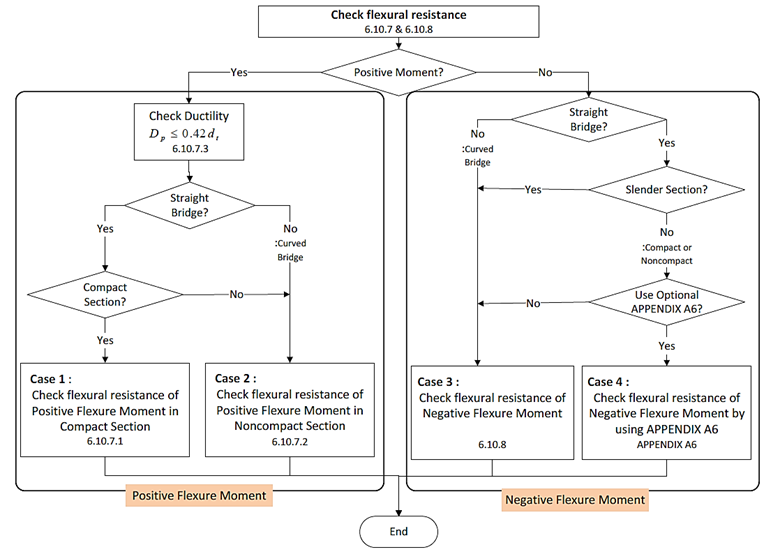Figure 1. Flowchart for calculation of Positive Moment Flexural Capacity as per AASHTO LRFD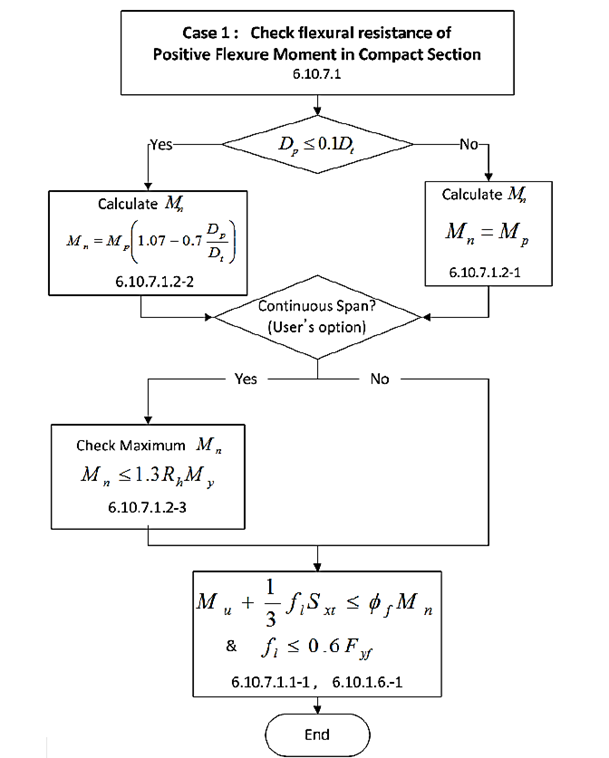Figure 2. Flowchart of the flexural resistance of Positive Flexure Moment in Compact Section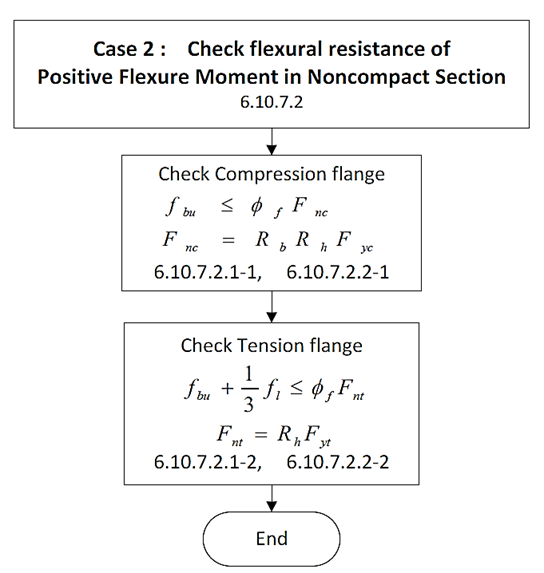Figure 3. Flowchart of the flexural resistance of Positive Flexure Moment in Noncompact Section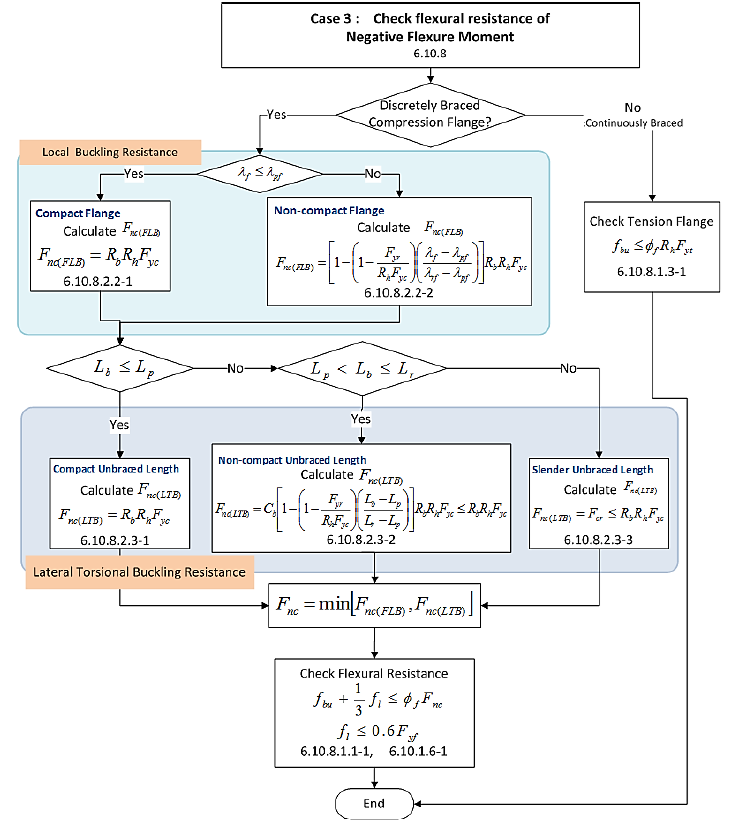Figure 4. Flowchart of the flexural resistance of Negative Flexure Moment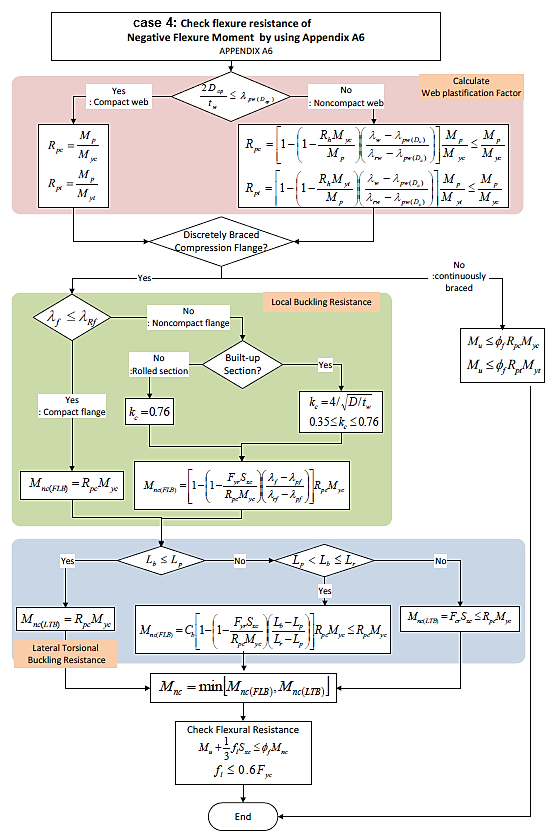Figure 5. Flowchart of the flexural resistance of Negative Flexure Moment by using Appendix A6

## 3. Flexural Resistance as per Eurocode

Bending resistance, MRd, can be calculated as follows based on its class.

Class 1 or 2 cross‐sections can be checked by using the plastic or elastic bending resistance.

Class 3 cross‐sections are checked with the elastic bending resistance, or possibly reclassified as effective Class 2 cross‐section and then checked with the plastic bending resistance.

Class 4 cross‐sections are also checked with the elastic bending resistance but by using the effective cross‐section, reduced to take account of buckling.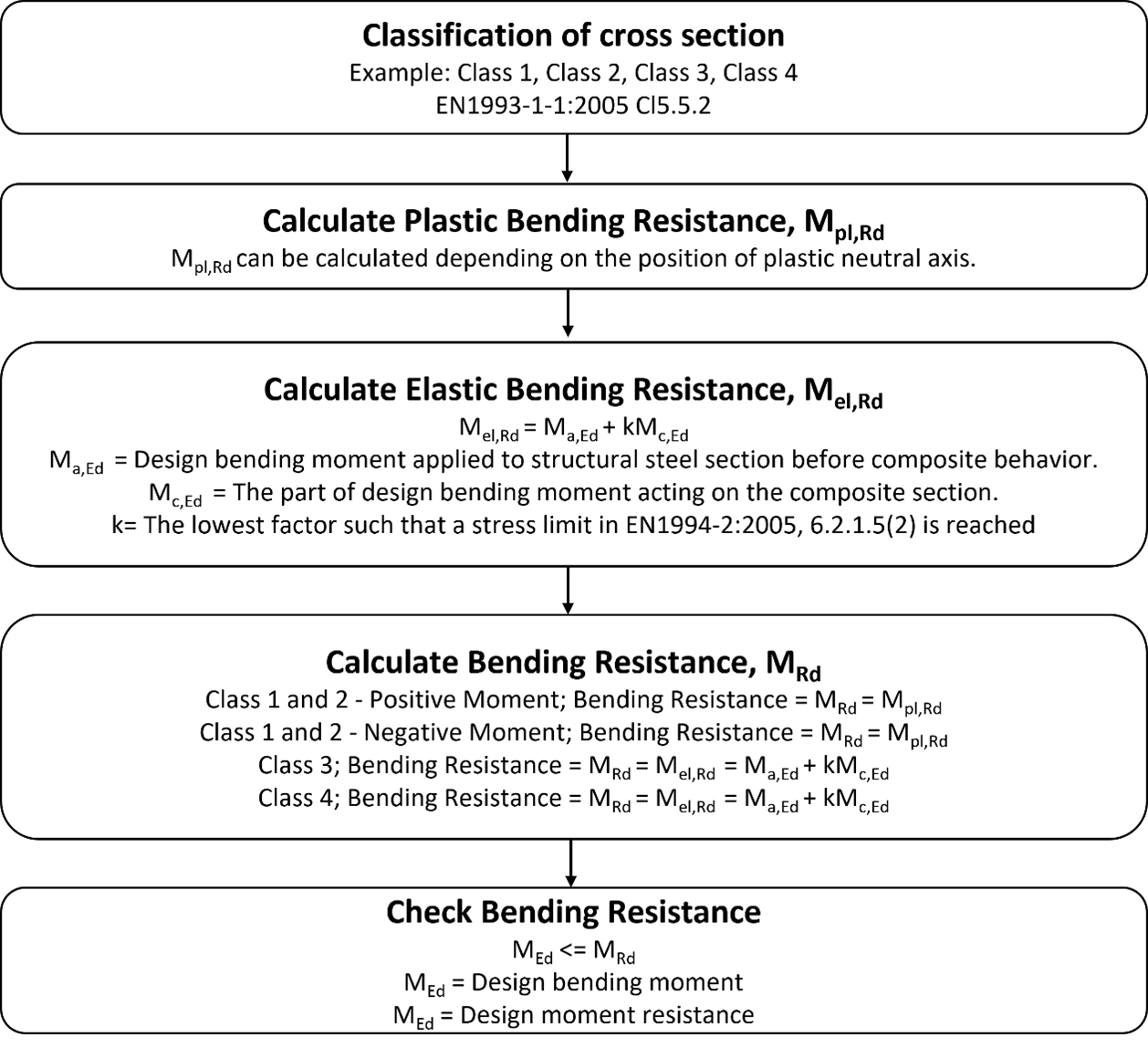Figure 6. Flowchart of flexural resistance as per Eurocode

## 4. Comparison of AAHSTO vs Eurocode Flexural Resistance

Let's take a 2-Span Steel Composite I girder Curved bridge for comparison.

Number of main girder: Four, Steel Composite I girder

Construction Stage Analysis: Yes

The bridge is modeled in midas Civil as shown below: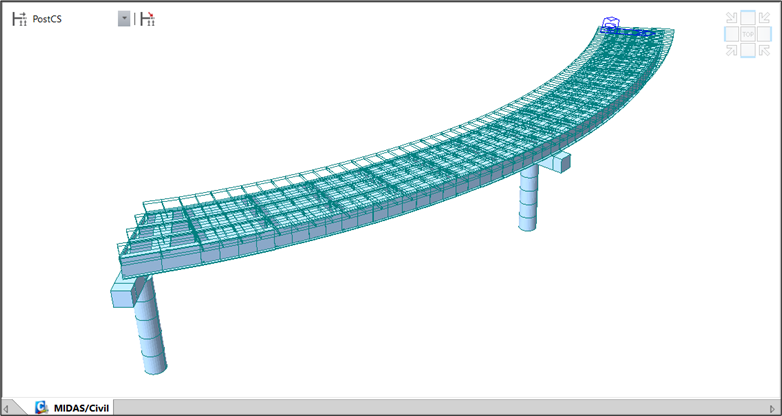Figure 7. Two-span Steel Composite I girder curved bridge model

Keeping the materials, sections, and loading the same, the positive bending region was designed as per AASHTO LRFD-17 and Eurocode (EN1994-2). The results are illustrated in figure 8 where the flexural resistance checks are satisfied as per the codes.

 AASHTO LRFD Eurocode SectionMaterials Steel       fsk= 355.000 MPa Es=210000.000    MPa          Concrete                                   fck= 30.000 MPa  Ecm = 33000.000 MPa           Reinforcement     fyk = 400.000 MPa Er = 210000.000 MPa Steel       fsk= 355.000 MPa Es=210000.000    MPa          Concrete                                   fck= 30.000 MPa  Ecm = 33000.000 MPa           Reinforcement     fyk = 400.000 MPa Er = 210000.000 MPa Region Positive Bending Positive Bending Demand Forces Steel only moment MD1 =2462 KNm   Long-term moment MD2 =1045 KNm   Short-term moment MD3 =1457 KNm Before Composite Moment Ma,Ed =2462 KNm   After Composite Moment Mc,Ed =2502 KNm   MEd = 4964 KNm Section Classification Non-compact section for Curved Bridge Class 1 Flexural Resistance ▪ Check Flexural Resistance of Composite noncompact section (AASHTO LRFD Bridge, 2018, 6.10.7.2)                                                i. Check compression flange             Fnc = Rb · Rh · Fyc = 355.000 MPa Fbu = 67.858 MPa              ≤ Фf · Fnc = 355.000 MPa HENCE OK                       ii. Check tension flange                      Fnt = Rh · Fyt = 355.000 MPa fbu + (1/3) fl = 99.115 MPa              ≤ Фf · Fnt = 355.000 MPa   HENCE OK                                            in which : Rb = 1.000 Rh = 1.000 Фf = 1.000 ▪ Check Flexural Resistance (EN 1994-2:2005)       - Plastic resistance moment, Mpl, Rd   Plastic NA = 1812.6 mm    Nslab  = 11221.020 kN                        Ng,top  = 5348.875 kN                          (Upper side of PNA)  Ng,bot =16569.895 kN                       (Lower side of PNA)    Mpl,Rd = 22842.225 kNㆍm           xpl = 330.327 mm                                                            MRd = βMpl,Rd = 22842.2kNㆍm                                     here, β =1.000                                                                                          MRd  = 22842.22 kNㆍm                      >MEd = 4965.21 kNㆍm HENCE OK
Figure 8. Flexural resistance comparison between AASHTO LRFD and Eurocode

## 5. Conclusion

The bending resistance of a steel composite girder can be calculated using the plastic stress distribution method. AASHTO LRFD uses load and resistance factor design where resistance factors(Фf) are multiplied with the actual bending resistance, whereas Eurocode uses partial safety factors for materials(γ) and reduction factors(β). The reduction factor β is applied only when high-strength steels of Fyk = 420 and 460 MPa are used.

In both the codes, based on section classification the moment capacity will be affected by the demand moments. So designer must prepare the load combinations in midas Civil carefully. AASHTO LRFD suggests moment resistance checks for compact sections in positive moment regions and for non-compact and negative bending regions, a stress check approach is performed. Whereas Eurocode suggests a moment resistance check approach only.